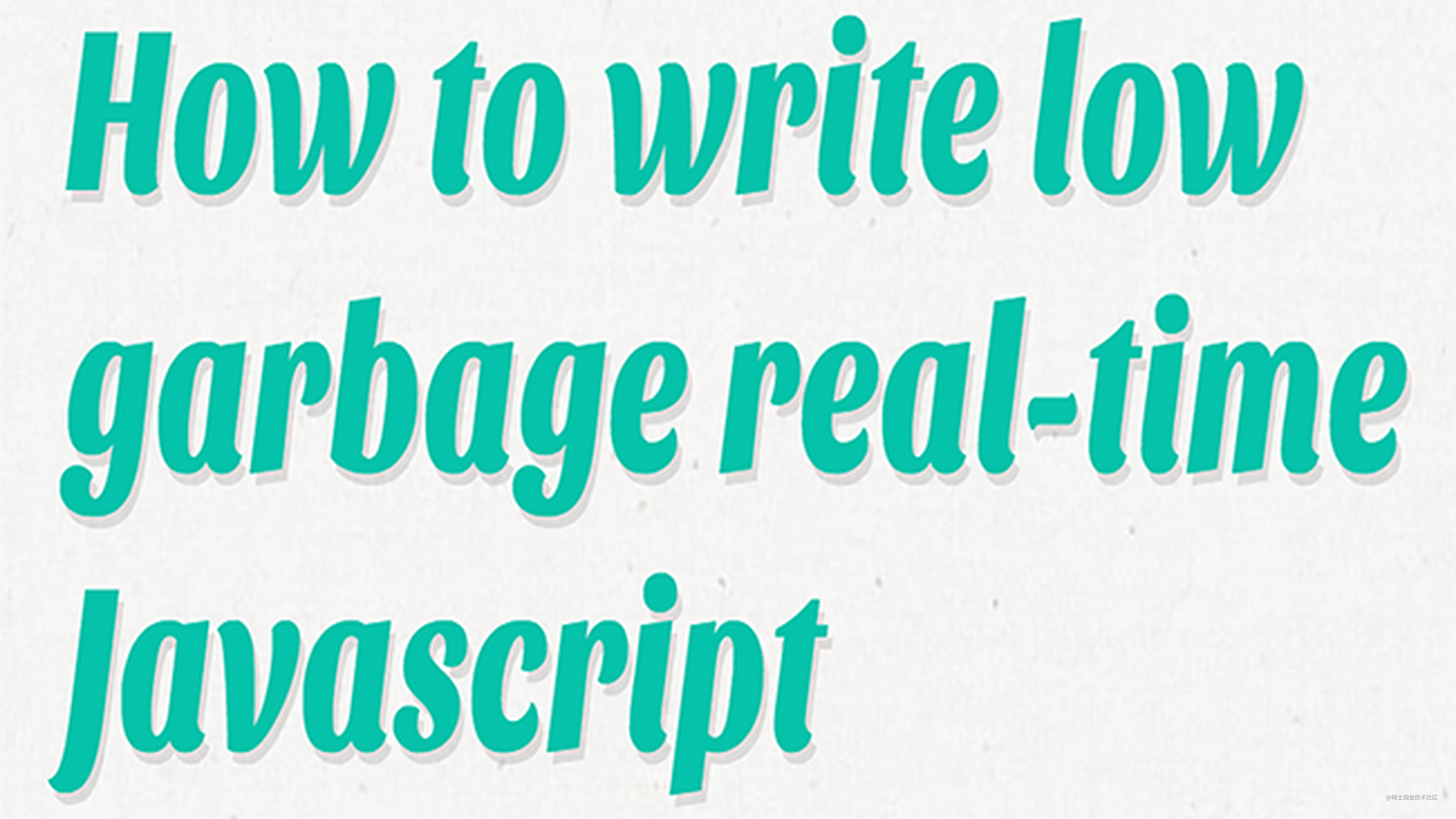# 如何编写避免垃圾开销的实时 Javascript 代码### 简单的技巧

`{}` (创建一个新对象)
`[]` (创建一个新数组)
`function () { ... }` (创建一个新函数，也会被垃圾收集)

``````// remove all own properties on obj,
effectively reverting it to a new object
cr.wipe = function (obj)
{
for (var p in obj)
{
if (obj.hasOwnProperty(p))
delete obj[p];
}
};
``````

```setTimeout((function (self) { return function () {
self.tick(); }; })(this), 16);```



``````// at startup
this.tickFunc = (function (self) { return function () {
self.tick(); }; })(this);

// in the tick() function
setTimeout(this.tickFunc, 16);
``````

### 进阶技巧

``````var sliced = arr.slice(index + 1);
arr.length = index;
arr.push.apply(arr, sliced);
``````

``````for (var i = index, len = arr.length - 1; i < len; i++)
arr[i] = arr[i + 1];

arr.length = len;
``````